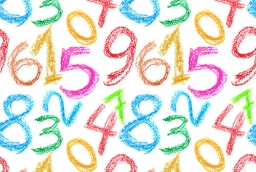# Determine 18233

Determine the unknown number for which it applies: whose four times increased by the number 3 equals its double

x =  -1.5

### Step-by-step explanation:

4x+3 = 2x

4·x+3 = 2·x

2x = -3

x = -3/2 = -1.5

x = -3/2 = -1.5

Our simple equation calculator calculates it.Did you find an error or inaccuracy? Feel free to write us. Thank you!

Tips for related online calculators
Do you have a linear equation or system of equations and looking for its solution? Or do you have a quadratic equation?